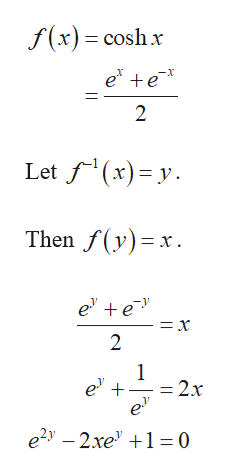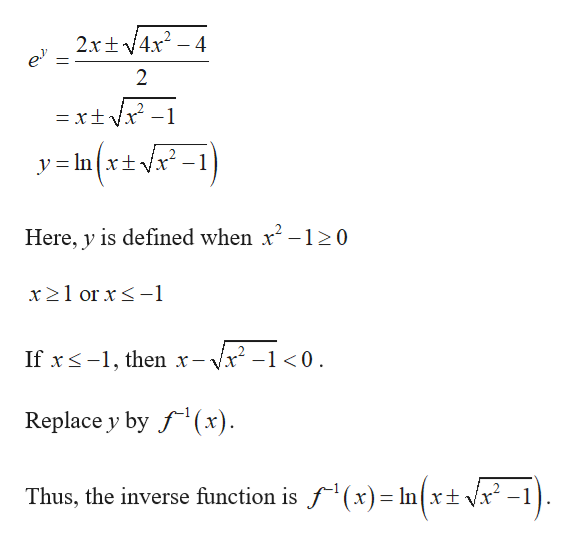# Q 9. (a) Find the inverse function of cosh(x). (Hint set z = e and invert that. Then replacee for z).(b) Find the derivative of cosh1 (x) using implicit differentiation and by differentiating thefunction you found in item (a).

Question
1 viewshelp_outlineImage TranscriptioncloseQ 9. (a) Find the inverse function of cosh(x). (Hint set z = e and invert that. Then replace e for z). (b) Find the derivative of cosh1 (x) using implicit differentiation and by differentiating the function you found in item (a). fullscreen
check_circle

Step 1

(a)Find the inverse function of cosh(x)help_outlineImage Transcriptionclosef(x) coshx e e x 2 Let f(x)y Then f(y)x e e = x 2 1 e 2x e e2y-2xe1= 0 fullscreen
Step 2

Solve the equ...help_outlineImage Transcriptionclose2xt4x2-4 2 = xt- y= In{x+vx -1 Here, y is defined when x2-120 x 21 or x1 If x-1 then x- /x2-10 Replace y by f(x) Thus, the inverse function is f(x)= In(xt Vx* -1 fullscreen

### Want to see the full answer?

See Solution

#### Want to see this answer and more?

Solutions are written by subject experts who are available 24/7. Questions are typically answered within 1 hour.*

See Solution
*Response times may vary by subject and question.
Tagged in

### Other